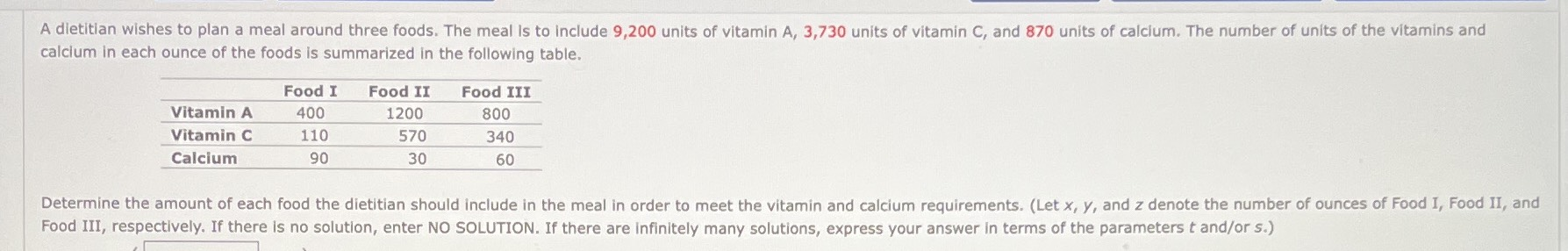### ¿Todavía tienes preguntas de matemáticas?

Pregunte a nuestros tutores expertos
Algebra
PreguntaA dietitian wishes to plan a meal around three foods. The meal is to include $$9,200$$ units of vitamin A, $$3,730$$ units of vitamin $$C$$ , and $$870$$ units of calcium. The number of units of the vitamins and calcium in each ounce of the foods is summarized in the following table.

Determine the amount of each food the dietitian should include in the meal in order to meet the vitamin and calcium requirements. (Let $$x , y ,$$ and $$z$$ denote the number of ounces of Food I, Food II, and Food III, respectively. If there is no solution, enter NO SOLUTION. If there are infinitely many solutions, express your answer in terms of the parameters $$t$$ and/or s.)